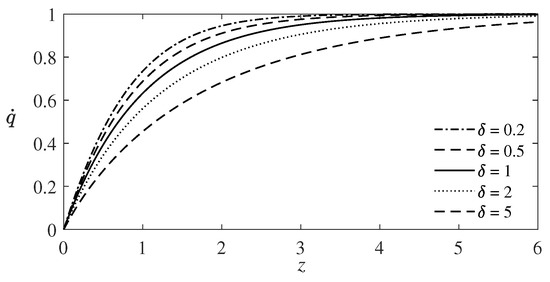Next Article in Journal
Remarks on Cosmological Bulk Viscosity in Different Epochs
Previous Article in Journal
Two-Phase Biofluid Flow Model for Magnetic Drug Targeting
Previous Article in Special Issue
Analytical Solutions to the Singular Problem for a System of Nonlinear Parabolic Equations of the Reaction-Diffusion Type
Open AccessFeature PaperArticle

# Group Analysis of the Boundary Layer Equations in the Models of Polymer Solutions

bySergey V. Meleshko 1,*andVladislav V. Pukhnachev 2,3
1
School of Mathematics, Institute of Science, Suranaree University of Technology, Korat 30000, Thailand
2
Lavrentev Institute of Hydrodynamics, Novosibirsk 630090, Russia
3
Department of Mechanics and Mathematics, Novosibirsk State University, Novosibirsk 630090, Russia
*
Author to whom correspondence should be addressed.
Symmetry 2020, 12(7), 1084; https://doi.org/10.3390/sym12071084
Received: 26 May 2020 / Revised: 6 June 2020 / Accepted: 11 June 2020 / Published: 1 July 2020
The famous Toms effect (1948) consists of a substantial increase of the critical Reynolds number when a small amount of soluble polymer is introduced into water. The most noticeable influence of polymer additives is manifested in the boundary layer near solid surfaces. The task includes the ratio of two characteristic length scales, one of which is the Prandtl scale, and the other is defined as the square root of the normalized coefficient of relaxation viscosity (Frolovskaya and Pukhnachev, 2018) and does not depend on the characteristics of the motion. In the limit case, when the ratio of these two scales tends to zero, the equations of the boundary layer are exactly integrated. One of the goals of the present paper is group analysis of the boundary layer equations in two mathematical models of the flow of aqueous polymer solutions: the second grade fluid (Rivlin and Ericksen, 1955) and the Pavlovskii model (1971). The equations of the plane non-stationary boundary layer in the Pavlovskii model are studied in more details. The equations contain an arbitrary function depending on the longitudinal coordinate and time. This function sets the pressure gradient of the external flow. The problem of group classification with respect to this function is analyzed. All functions for which there is an extension of the kernels of admitted Lie groups are found. Among the invariant solutions of the new model of the boundary layer, a special place is taken by the solution of the stationary problem of flow around a rectilinear plate. View Full-Text
Show FiguresFigure 1

MDPI and ACS Style

Meleshko, S.V.; Pukhnachev, V.V. Group Analysis of the Boundary Layer Equations in the Models of Polymer Solutions. Symmetry 2020, 12, 1084.